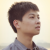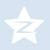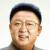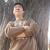## 一组不规则的数字拼成一个固定的数字，求指点

1``````public class TenThousand {

public static void main(String[] args) {
Integer[] numbers = new Integer[]{4000, 7000, 2500, 3500, 3500, 2000, 1000, 4500, 3500, 6000, 8000, 1000};
List<Integer> numberList = new ArrayList<Integer>(Arrays.asList(numbers));
System.out.println("array : " + numberList);

Random random = new Random();

int sum = 0;
Map<Integer, Integer> select = new HashMap<Integer, Integer>();
while (true) {
int i = random.nextInt(numberList.size());
if (select.containsKey(i)) {
continue;
}
select.put(i, numberList.get(i));
sum += numberList.get(i);

if (sum == 10000) {
System.out.println("equal 10000 index:" + select.toString());
for (int s : select.keySet()) {
System.out.println("remove value : " + select.get(s));
numberList.remove(select.get(s));
}
sum = 0;
select.clear();
System.out.println("new array : " + numberList);
} else if (sum > 10000) {
//                System.out.println("greater than 10000:" + select.toString());
sum = 0;
select.clear();
}
}

}
}
``````

1for(剩下得数)

{

if(>10000){

}else{

}}000